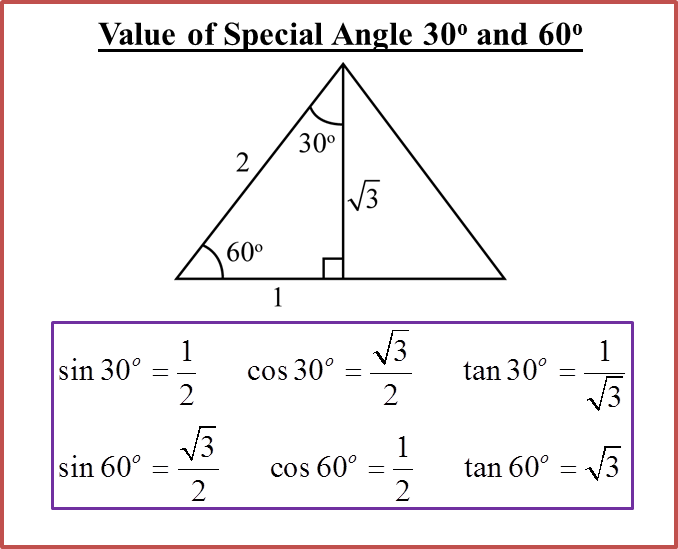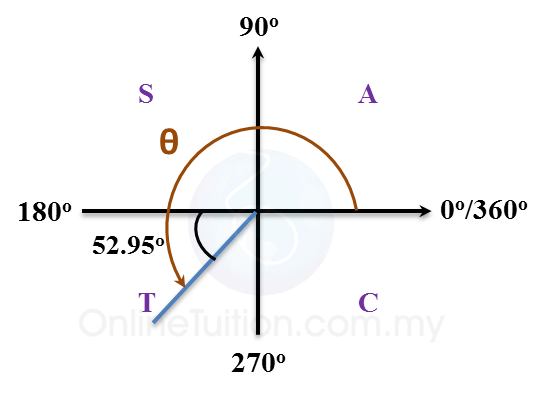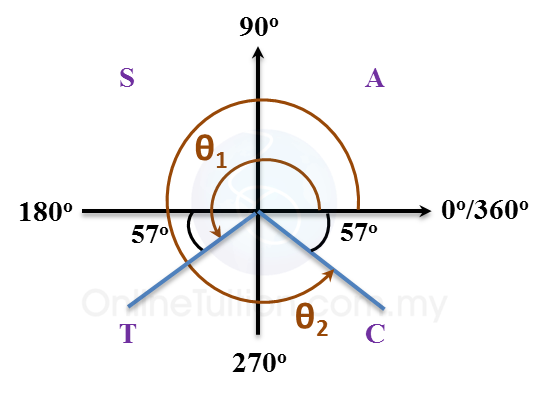# 9.1.2 Values of Sine, Cosine and Tangent of an Angle (Part 2)

(A) Special Angle(B) Finding the Angles between 0oand 360o Given Values of sin θ, cos θ or tan θ

1. If the value of sin θ, cos θ or tan θ is given and 0o< θ < 360o, the value of θ can be found using the following steps.

(a) Find the basic angle in first quadrant which corresponds to θ.

(b) Based on the sign of the value of sin θ, cos θ or tan θ, determine the quadrants in which θ lies.

(c) Find the values of θ in the right quadrants found in (b).

Example:
For each of the following cases, find the value of θ.
(a) sin θ = 0.6025 and 90o < θ < 180o
(b) cos θ = –0.6025 and 180o < θ < 270o
(c) sin θ = –0.8387 and 0o < θ < 360o
(d) tan θ = –1.732 and 0o < θ < 360o

Solution:
(a)sin θ = 0.6025
Basic ∠ = 37.05o ← (Press SHIFT sin-1 0.6025 =Display: 37.04976)
∴ θ = 180o – 37.05= 142.95o  ← (90o < θ < 180o)

(b)cos θ = –0.6025
Basis ∠ = 52.95o ← (Press SHIFT cos-1 0.6025 = Display: 52.9508)
∴ θ = 180o + 52.95= 232.95o  ← (180o < θ < 270o)

(c)sin θ = –0.8387 ← (sin θ is negative in 3rd quadrant and 4thquadrant)
Basis ∠ = 57o ← (Press SHIFT sin-1 0.8387 = Display: 57.003)
θ1 = 180o + 57o = 237o  ← (180o < θ < 270o)
θ2 = 360o – 57o = 303o  ← (270o < θ < 360o)
θ = 237o and 303o

(d)tan θ = –1.732 ← (tan θ is negative in 2nd quadrant and 4thquadrant)
Basis ∠ = 60o ← (Press SHIFT tan-1 1.732 = Display: 59.9993)
θ1 = 180o – 60o = 120o  ← (90o < θ < 180o)
θ2 = 360o – 60o = 300o  ← (270o < θ < 360o)
θ = 120o and 300o